TO THE WEST.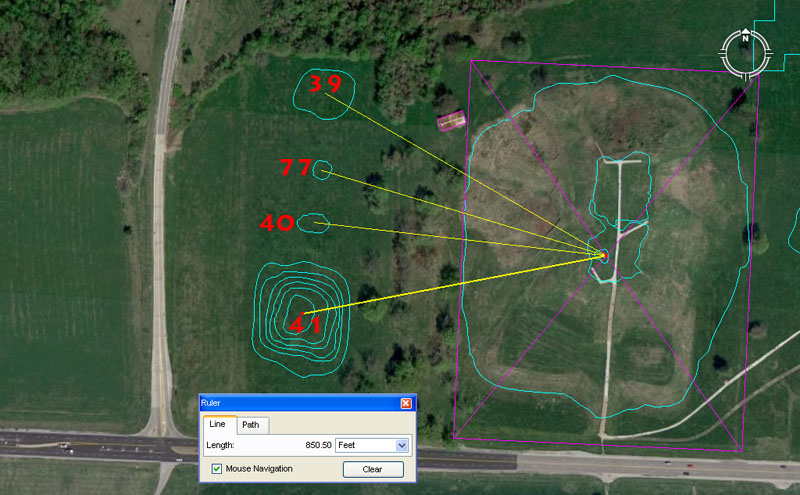Mounds 39, 40 & 41 sit on the western side of Monk's Mound, but so does mound 77. Page 155 of The Cahokia Atlas, A Historical Atlas of Cahokia Archaeology, by Melvin Fowler states: 'On Moorehead's 1929 map, four mounds—39, 77, 40, and 41—form a line paralleling the west edge of Monks Mound (Figure 6.1). Mound 77 is Moorehead's addition to this alignment, with 39, 40, and 41 previously noted clearly on the Patrick and other maps.'

Mound 39 sits 891' from the hub of Monk's Mound at an azimuth angle of 300-degrees. This seemingly strange mathematical coding recurs on ancient open-air-university sites from Avebury and Durrington Walls henges in England to Ring o' Brodgar in Scotland to Nazca in Peru to Easter Island and, thus, had very obvious importance to ancient navigators. It is part of an "11" progression (11 X 9 X 9 = 891) and 24750 (the value used to describe the equatorial circumference under the "11" system) X 36 = 891000. Also, the Scottish mile @ 5940' X 15 = 89100.

The 300-degrees angle out to this mound is 5/6ths of 360-degrees.

Mound 77, which is a part of this group, sits 816.75' from the hub position on Monk's mound at a return angle of 106.66666-degrees. In the 130680000' circumference ("11" system) of the Earth the sum of 8167500' would be 1/16th part. A much used value in mathematical progressions for coding the equatorial circumference is based upon the value 6534 of which 816.75 is 1/8th part.

The sum of 106.66666 is 1/3rd of 400. As mentioned the old French foot was 1.066666' or 12.8" and the mathematical progression had very obvious importance to ancient mathematicians.

Mound 40 sits a coded or intended distance of 809.01695' @ 276.48-degrees from the hub position atop Monk's Mound. This position would have been equally read as 810'. The tutorials here would have been about the calculating merits of PHI (1: 1.6180339 and rounded PHI 1: 1.62), which were also used as half values (.80901696 & .81). At English sites, like Stonehenge, there are multiple concentric circles of posts or standing stones. The post circles would reduce in diameter by PHI. Nevertheless, ancient mathematicians would overlay a circle with a square and the square would be a half-PHI reduction on the diameter of the circle. This occurred at Stonehenge.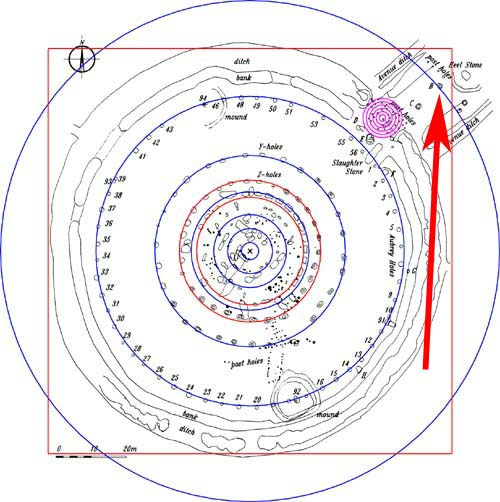The red arrow points to a marker situated on the Avenue at Stonehenge, which sits 233.28' (also read as 233.3333') from the centre of Stonehenge. From this position the main circles of Stonehenge (with exception to "Z" Holes and the inner rim of the Sarsen Circle ...shown in red) reduce by the PHI ratio of 1: 1.6180339. It can be observed that a half-PHI (.80901695) reduced square overlaying the 466.56 diameter circle contains the Stonehenge site's circular perimeter (378' wide... East to West), with only some Avenue markers (like the Heel Stone) jutting beyond. Therefore, at Stonehenge the PHI reduction (slightly rounded) for the circles go: 466.56' to 288' (Aubrey Circle) to 178.2 ("Y" Holes) to 110' (outer rim of the Sarsen Circle). Each of these numbers is highly coded and factorable and recur in ancient European/Mediterranean Weights, Measures & Volumes standards. The slightly rounded PHI reducing numbers can also be represented as 466 - 288 = 178; 288 - 178 = 110.

The point is that, at Cahokia Mounds, the tutorials were the same as elsewhere at open-air-universities across the world and the PHI ratio, its half value and reciprocal value were taught. The PHI reciprocal was especially important to know when fabricating round volume vessels for dispensing exact cubic inch volumes in the marketplace. In a sense, the PHI reciprocal allowed ancient merchants to "square the circle".

The angle out to mound 40 is 276.48-degrees and 276.48-miles is 1/90th of the 24883.2-mile equatorial circumference.

Mound 41 has dynamic coding to do with the duration of the lunar nutation cycle and the Precession of the Equinoxes. It sits a coded distance of 850.5' from the Monk's Mound hub @ 259.2-degrees.

With the lunar nutation cycle enduring for 6804-days, 1/8th of that period is 850.5-days. Mound 41 sits half the distance out as mound 30 (1701') and a mathematical progression based upon 850.5 carries the same lunar cycle information.

The angle relates to the Precession of the Equinoxes, which ancient mathematicians calculated (in factorable numbers) to move 1-degree each 72 years. Therefore 72 X 360 = 25920-years for the full cycle of Precession. It is obligatory to find expressions of 2592 encoded into the major, ancient open-air-universities.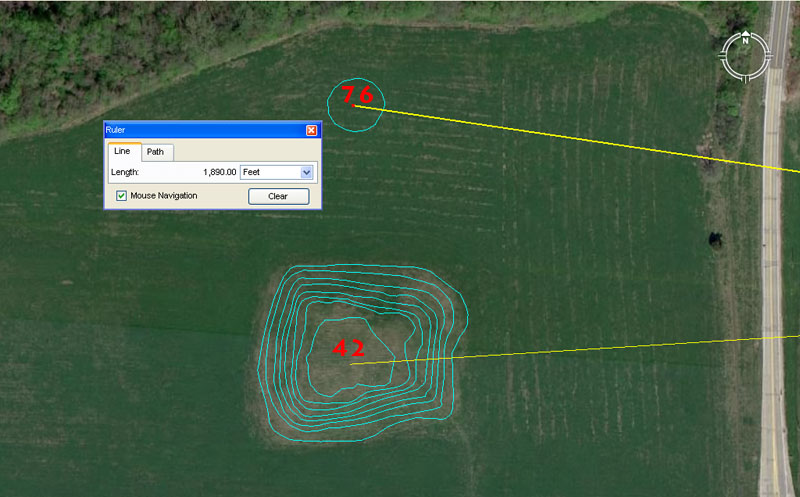Mound 42 sits 1875' from the hub on Monk's Mound at an azimuth angle of 86.4-degrees return. Nearby is mound 76 and it is convenient to include its coding at the same time.

Mound 42 is very substantial and, up until recent decades, had large buildings on top of it and to its eastern side. The number 1875, placed in a mathematical progression, will generate multiple values that were very useful in navigation, the compass, lunar calculations. The sum of 187.5' would be 1/28th of a Greek mile and 1/88th of an English league of 16500'.

The 86.4-degrees angle value is part of a very important multi-use mathematical progression that goes 864 (the Sun is 864,000-miles in diameter), 1728 (note a cubic foot @ 12 X 12 X 12" is 1728 cubic inches). Note also that Silbury Hill of Southern England has a circumference of 1728', based upon a diameter of 550' and this relationship between an "11" series number converting to a circumference that was perfectly divisible within a 360-degree environment, was the mathematical basis of positional-plotting at sea. The rendition of PI used for converting "11" series diameters into perfect circumferences was 1728/550ths. (3.141818182). The progression continues with: 2592 (Precession), 3456 (note: the outer rim of the Sarsen Circle at Stonehenge measured 345.6'), 4320 (note: The Great Pyramid is 756' per side or 432 Celtic / Hebrew cubits of 21" each), 5184 (note the slope angle of the Great Pyramid is 51.84-degrees - 5184-years is 1/5th of the 25920-year Precessional cycle - The Great Pyramid @ 756' per side is 72 Reed of 10.5' each per side and covers an area of 5184 square reeds- An English league diameter @ 16500' converts to a circumference of 51840' using PI @ 1728/550ths) etc.

This is the kind of information that prospective navigators in training had to memorise in order to develop minds that worked like calculators. The sea does not abide fools well and insufficiently trained navigators were probably going to die, along with the crew under their uncertain guidance.

Mound 76 sits 1890' out from Monk's Mound hub @ 98.4375-degrees (987/16ths) return. The Great Pyramid @ 756' long is 189' X 4. The day count on the ancient Druidic Calendar of Coligny parapegma brass calculator was 1890. The count was either 63 solar months X 30 = 1890-days or 64 lunar months (29.53125-days) = 1890-days. The return degree angle is lunar and 9.84375-days would be 1/3rd of a lunar month or 1/36th of a lunar year.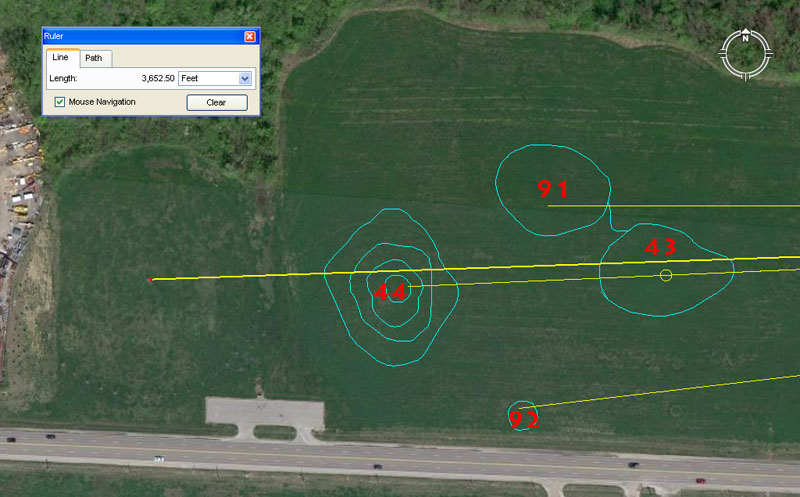Again, because of the somewhat erratic numbering system assigned to the Cahokia Mounds, numbers 91 & 92 are included here with mounds 43 & 44. Also, to the left side of the picture the circular aspect of Cahokia's Woodhenge post circle can be vaguely detected.

Mound 43 sits 2750' from the hub atop Monk's Mound @ a return angle of 87.5-degrees. The sum of 2750' is 1/6th of an ancient English league of 16500' (3.125-miles), whereas the sum of 875' (based upon the coded degree angle) would be 1/6th of a Greek mile of 5250'.

The sum of 2750-miles would be 1/9th of the 24750-mile equatorial circumference ("11" system of navigation).

Mound 91 sits a coded distance of 2953.125' from the Monk's Mound hub @ an azimuth angle of 270-degrees (due West). The 2953.125' distance is, of course, in homage to the 29.53125-days in a lunar month. There would also have been a reading of 2600' to accentuate "26" or 13 X 2. Under the Sabbatical Calendar system there were either 13 months in the year or 52 weeks of 28-days each, with a half year of 26-weeks (the period between equinoxes).

A post marker atop mound 91 would, undoubtedly, have marked due West, such that student navigators at the hub position on Monk's Mound could set their manual theodolites to get perfect angle readings onto all other targets around the horizon.

Mound 92 sits 3024' out at a return azimuth angle of 83.33333-degrees. The distance is, of course, half a minute of equatorial arc (6048') under the 24883.2 Greek mile navigational system encoded, very literally, into the base dimensions of the Great Pyramid and founded upon the "6&7"number families combined. The tutorial would also have included the value 3025' for teaching the "11" family system of navigation and positional plotting at sea. Under that system the Earth was configured to be 24750-miles in equatorial circumference and half a minute of arc was 3025'.

The return angle of 83.33333 sets up a mathematical progression that goes: 83.33333, 166.66666, 250, etc. As mentioned,, in a 3,4,5, triangle the short side will be 1.66666 less that the hypotenuse side. The 1.66666 value (12/3rds) appears to have been used copiously in ancient calculations.

Mound 44 sits 3200' out at the same azimuth angle as mound 43. The 360-degree calendar is read in both 36 & 32 divisions simultaneously, with the 32 division reading providing the primary and secondary points of the compass. The coded distance to the crown position of mound 44 would also have included a tutorial about 3240', as a mathematical progression based upon this number provides very important multi-use values.

Woodhenge's centre post sits 3652.5' from the hub position atop Monk's Mound at a return azimuth angle of 88-degrees. The distance is in homage to the 365.25-days in a solar year. The very clever way in which the ancient astronomers set the centre post of Cahokia's Woodhenge circle, meant that first glint of the equinoctial sunrise would be observed where the base of the 3rd terrace met the top of the 2nd terrace. This notch on Monk's Mound sat very close to 91.3125-degrees and the sum of 91.3125-days is 1/4th of a solar year of 365.25-days or 1/2 of 182.625-days (the number of days between equinoxes).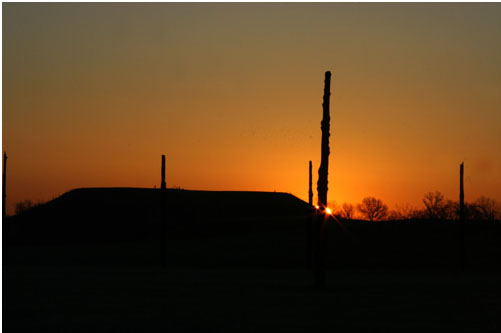From the centre post of Cahokia's Woodhenge. the Sunrise on the day of the equinox was coded to represent 91.3125-degrees azimuth as the rise position. (Photo by Scott McNealy).

Continue